# Exercise E4.3 Linear Equations in Two Variables NCERT Solutions Class 9

Go back to  'Linear Equations in Two Variables'

## Chapter 4 Ex.4.3 Question 1

Draw the graph of each of the following linear equations in two variables:

(i) $$x + y = 4$$

(ii) $$x – y = 2$$

(iii) $$y = 3x$$

(iv) $$3 = 2x + y$$

### Solution

What is known?

Linear equations

What is Unknown?

How to draw graph

Reasoning:

First of all, we can draw table for different values of $$x$$ and $$y$$ and then with the help of the values we can plot graph for each equation.

Steps:

(i) $$x + y = 4$$

Re-write the equation as

\begin{align}&x + y = 4 \\&\Rightarrow y = 4 \,– x \quad\dots\text{Equation } (1)\end{align}

By substituting the different values of $$x$$ in the Equation (1), we get different values for $$y$$

• When $$x = 0,$$ we have: $$y = 4 – 0 = 4$$
• When $$x = 2,$$ we have: $$y = 4 – 2 = 2$$
• When $$x = 4,$$ we have: $$y = 4 – 4 = 0$$

Thus, we have the following table with all the obtained solutions:

 $$x$$ $$0$$ $$2$$ $$4$$ $$y$$ $$4$$ $$2$$ $$0$$

By Plotting the points $$(0, 4) (2, 2)$$ and $$(4, 0)$$ on the graph paper and drawing a line joining the corresponding points, we obtain the Graph.

The graph of the line represented by the given equation is as shown.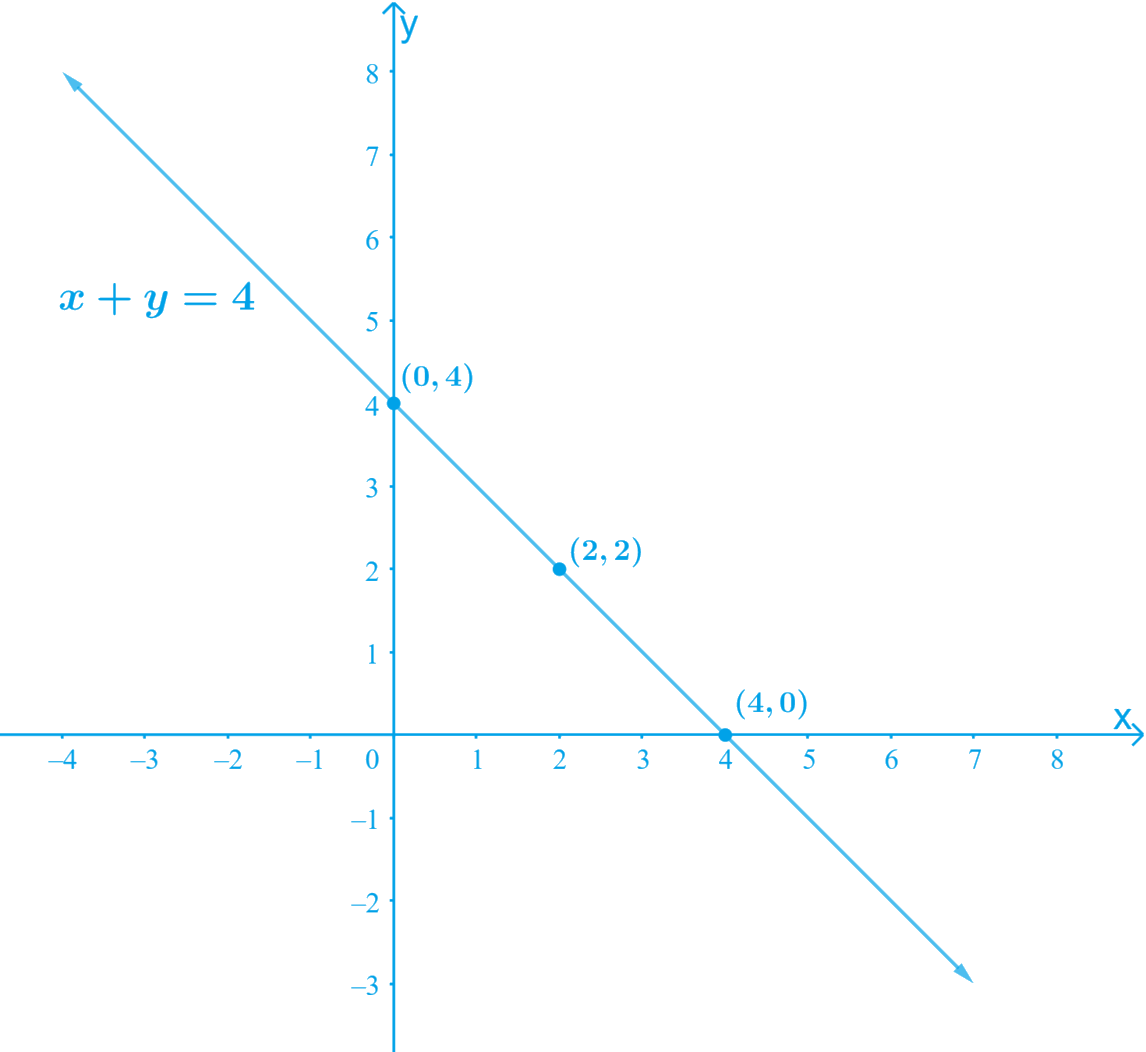(ii) $$x – y = 2$$

Re-write the equation as
\begin{align}&x - y = 2\\&\Rightarrow y = x \,– 2\; \quad \dots\text{Equation } (1)\end{align}

By substituting the different values of $$x$$ in the Equation (1) we get different values for $$y$$

• When $$x = 0,$$ we have $$y = 0\, – 2 =\, – 2$$
• When $$x = 2,$$ we have $$y = 2 \,– 2 = 0$$
• When $$x = 4,$$ we have $$y = 4 \,– 2 = 2$$

Thus, we have the following table with all the obtained solutions:

 $$x$$ $$0$$ $$2$$ $$4$$ $$y$$ $$-2$$ $$0$$ $$2$$

By Plotting the points $$(0, –2)$$, (2, 0) and (4, 2) on the graph paper and drawing a line joining the corresponding points, we obtain the Graph.

The graph of the line represented by the given equation is as shown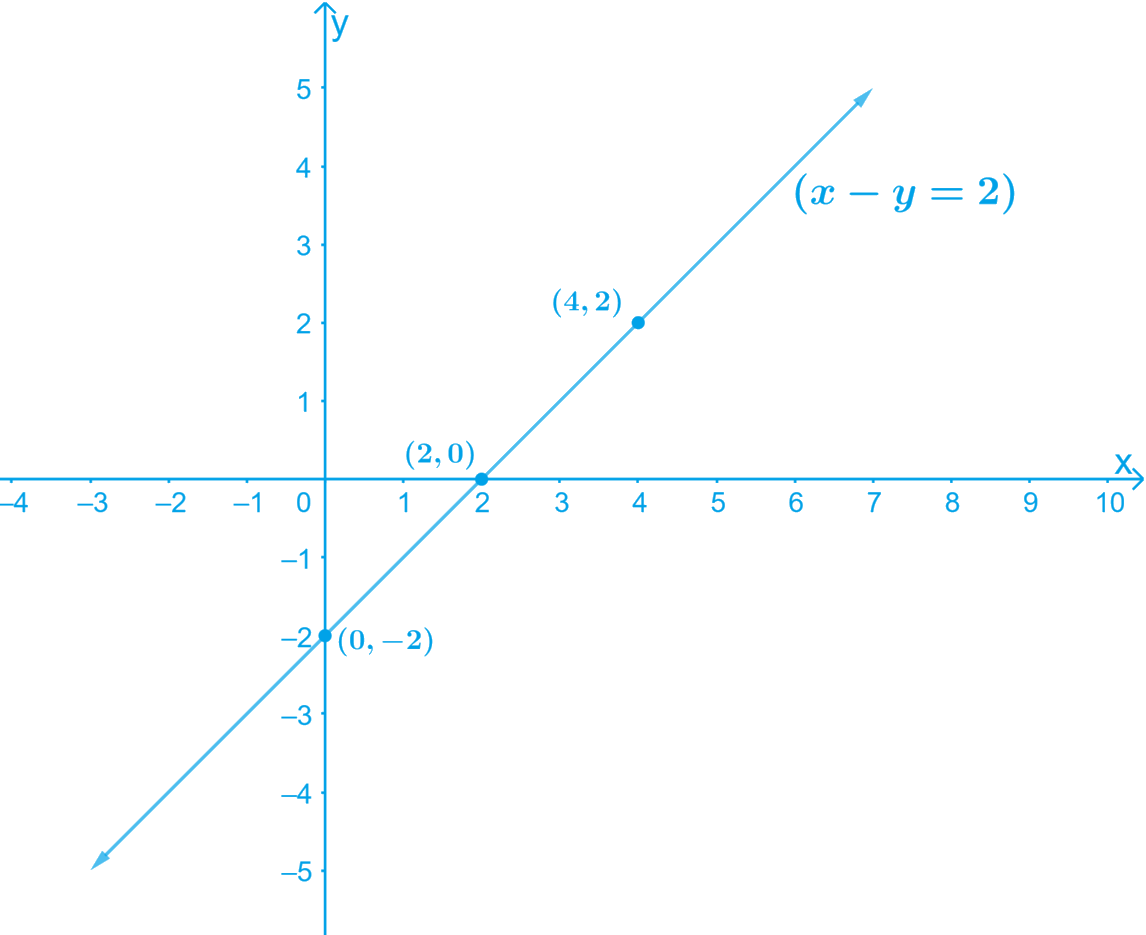(iii) $$y = 3x$$

By substituting the different values of $$x$$ in the Equation (1) we get different values for $$y$$

• When $$x = 0,$$ we have: $$y = 3(0) = 0$$
• When $$x = 1,$$ we have: $$y = 3 (1) = 3$$
• When $$x =\,–1,$$ we have:

$$y = 3(–1) = \,– 3$$

Thus, we have the following table with all the obtained solutions:

 $$x$$ $$0$$ $$1$$ $$-1$$ $$y$$ $$0$$ $$3$$ $$-3$$

By Plotting the points $$(0, 0), (1, 3)$$ and

$$(–1, –3)$$ on the graph paper and drawing a line joining the corresponding points, we obtain the Graph.

The graph of the line represented by the given equation is as shown: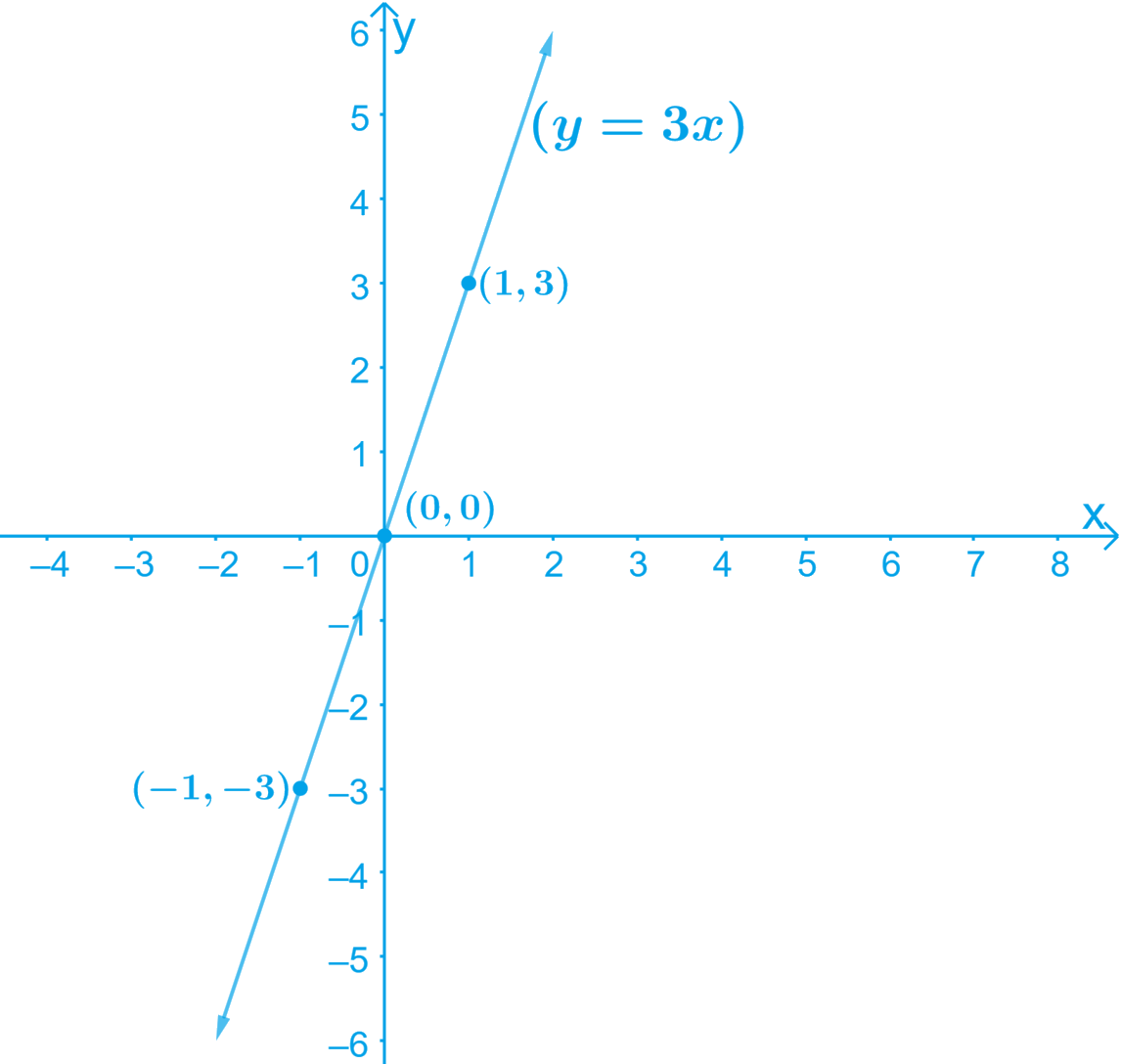(iv) $$3 = 2x + y$$

Re-write the equation as
\begin{align} y = 3 – 2x\; \quad \dots\text{Equation } (1)\end{align}

By substituting the different values of $$x$$ in the Equation (1) we get different values for $$y$$

• When $$x = 0,$$ we have:

$$y = 3 \,– 2(0) = 3 \,– 0 = 3$$

• When $$x = 3,$$ we have:

$$y = 3 \,– 2(3) = 3 \,– 6 = \,– 3$$

• When $$x = \,– 1,$$ we have:

$$y = 3 \,– 2 (–1) = 3 + 2 = 5$$

Thus, we have the following table with all the obtained solutions:

 $$x$$ 0 3 $$-1$$ $$y$$ 3 $$-3$$ 5

By Plotting the points $$(0, 3)$$, $$(3, –3)$$ and $$(–1, 5)$$ on the graph paper and drawing a line joining the corresponding points, we obtain the Graph.

The graph of the line represented by the given equation is as shown.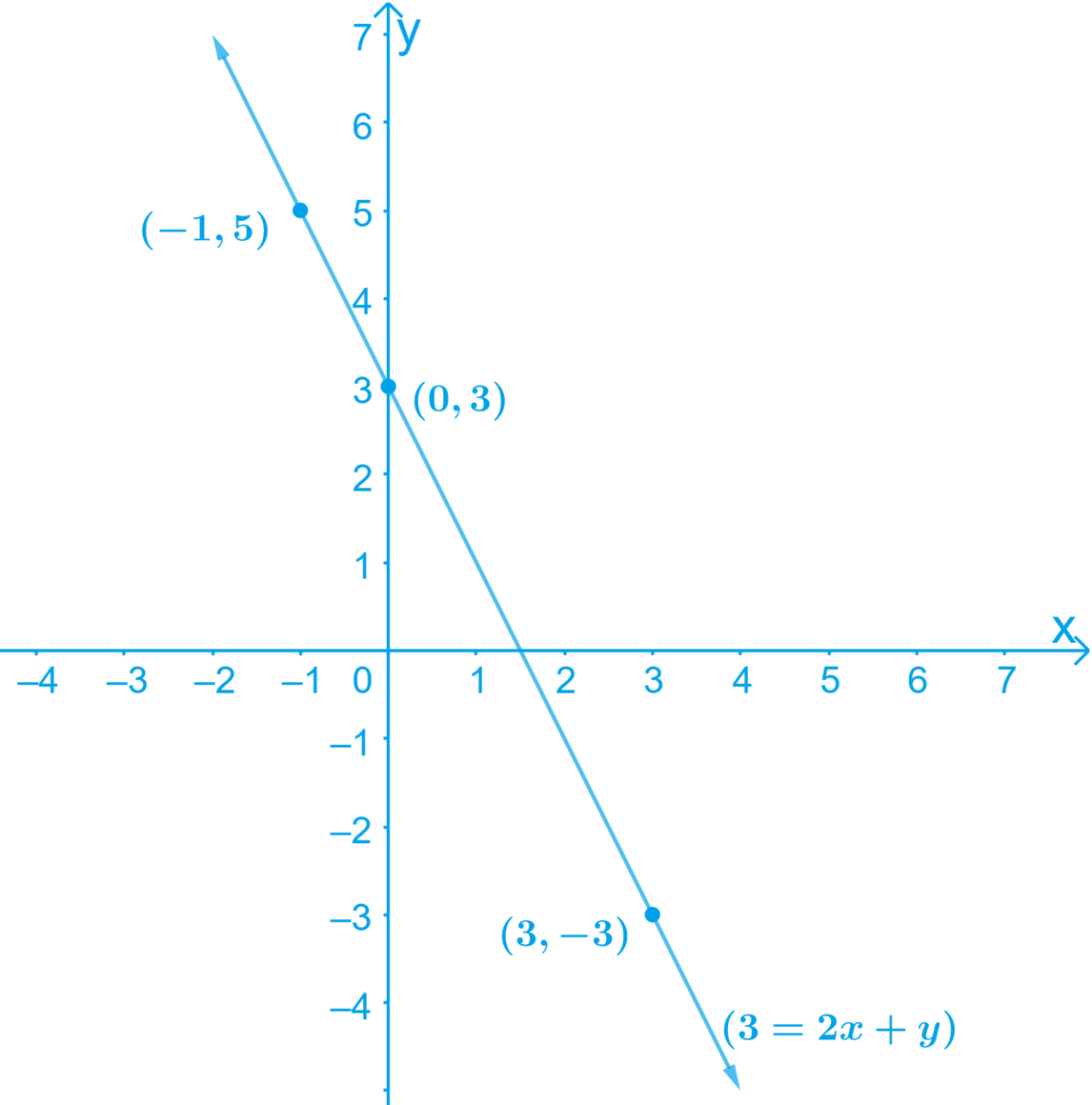## Chapter 4 Ex.4.3 Question 2

Given the equations of two lines passing through $$(2, 14).$$ How many more such lines are there, and why?

#### ### Solution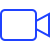Video Solution

What is known?

Point ($$2, 14$$) at which line passes through

What is Unknown?

Equation of line and number of lines which can passes through the given point.

Reasoning:

We can think for those equations which could satisfy the given point.

#### Steps:

It can be observed that point $$(2, 14)$$ satisfies the equation $$7x − y = 0$$ and $$x − y + 12 = 0$$.

Therefore, $$7x − y = 0$$ and $$x − y + 12 = 0$$

are two lines passing through point $$(2, 14).$$

As it is known that through one point, infinite number of lines can pass through, therefore, there are infinite lines of such type passing through the given point.

## Chapter 4 Ex.4.3 Question 3

If the point $$(3, 4)$$ lies on the graph of the equation $$3y = ax + 7$$, find the value of $$a$$.

### Solution

What is known?

Linear equation $$3y = ax + 7.$$

What is Unknown?

Value of $$a$$.

Reasoning:

We can find value of $$a$$ by substituting the values of $$x$$ and $$y$$ in the given equation

#### Steps:

Given :

$$3y = ax + 7$$ is the Linear Equation $$\quad \dots\text{ Equation} (1)$$

Point $$(3, 4)$$ lies on the Equation (1)

By Substituting the value of $$x = 3$$ and $$y = 4$$ in the Equation ($$1$$),

\begin{align} 3 y & = a x + 7 \\ 3 (4) & = a (3) + 7 \\12 \,\,– 7&=3a \\5 & = 3 a \end{align}

Hence, the value \begin{align} a = \frac { 5 } { 3 }\end{align}

## Chapter 4 Ex.4.3 Question 4

The taxi fare in a city is as follows: For the first kilometer, the fare is $$₹\, 8$$ and for the subsequent distance it is $$₹\, 5 \,\rm{per \,km.}$$ Taking the distance covered as $$x$$ $$\rm{km}$$ and total fare as $$₹$$ $$y$$, write a linear equation for this information, and draw its graph.

### Solution

What is known?

Distance covered is $$x$$ $$\rm{km}$$ and total fare is $$₹$$. $$y$$. Also fare of first kilometer is $$₹\, 8$$ and for subsequent distance is $$₹\, 5$$

What is Unknown?

Linear equation for given condition and also graph of it.

Reasoning:

We know fare for first $$\rm{km}$$ and add the fare of subsequent distance and the result will form linear equation. After that by finding the solution for linear equation we can draw graph of it.

#### Steps:

Given,

Let the Total distance covered $$= x\,\rm{km}$$

Let the Total fare covered $$= ₹$$ $$y$$

Therefore, subsequent distance $$= (x -1) \rm{km}$$

Fare for the subsequent distance $$= ₹ 5 (x − 1)$$

Fare for $$1$$st kilometer $$= ₹\, 8$$

The linear equation for the above information is given by,

Total fare

\begin{align} &(y) = [ 8 + ( x - 1 ) 5 ] \\ &y = 8 + 5 x - 5 \\ &y = 5x + 3\\ &5 x - y + 3 = 0 \end{align}

Re-write the equation by transposing as $$y = 5x + 3 \qquad \dots \dots \text{ Equation } (1)$$

By substituting different values of $$x$$ in the Equation (1) we get different values for $$y$$

• When $$x = 0$$,

$$y = 5 × 0 + 3 = 0 + 3 = 3$$

• When $$x = 1$$,

$$y = 5 × (1) + 3 = 5 + 3 = 8$$

• When $$x = 2$$,

$$y = 5 × (2) + 3 = 10 + 3 = 13$$

• When $$x = -1$$,

$$y = 5 × (–1) + 3 =\, –5 + 3 =\, -2$$

• When $$x = -2$$,

$$y = 5 × (–2) + 3 =\, –10 + 3 =\, -7$$

Thus, we have the following table with all the obtained solutions:

 $$x$$ $$0$$ $$1$$ $$2$$ $$-1$$ $$-2$$ $$y$$ $$3$$ $$8$$ $$13$$ $$-2$$ $$-7$$

By Plotting the points $$(0, 3), (1, 8), (2, 13),$$ $$(-1, -2), (-2, -7)$$ on the graph paper and drawing a line joining them, we, obtain the required graph

The graph of the line represented by the given equation as shown: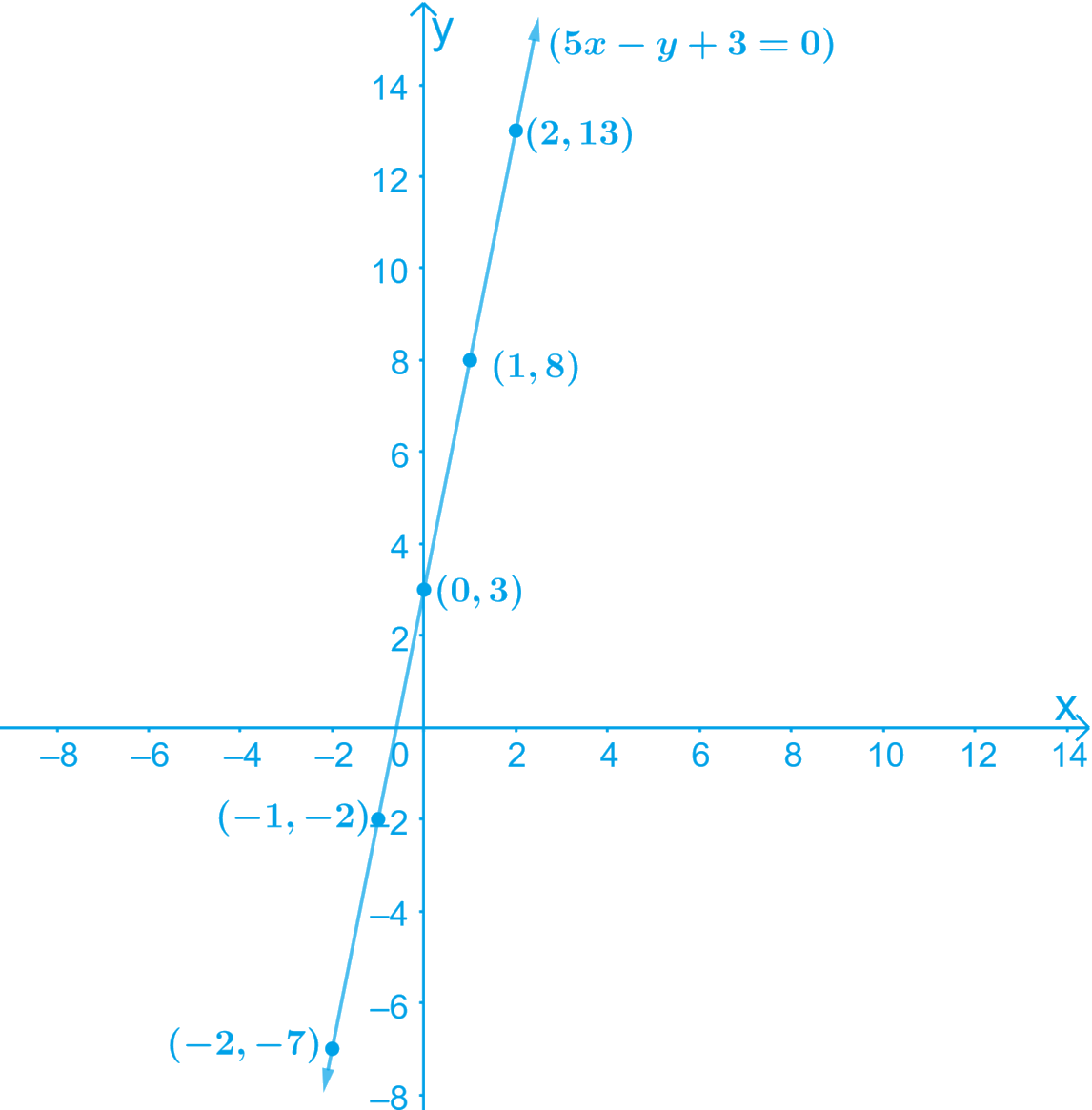Here, the variable $$x$$ and $$y$$ are representing the distance covered and the fare paid for that distance respectively and these quantities may not be negative.

Hence, only those values of $$x$$ and $$y$$ which are lying in the $$1\rm st$$ quadrant will be considered.

## Chapter 4 Ex.4.3 Question 5

From the choices given below, choose the equation whose graphs are given in the following figures.

 For the first figure For the second figure (i) $$y = x$$ (i) $$y = x + 2$$ (ii) $$x + y = 0$$ (ii) $$y = x − 2$$ (iii) $$y = 2x$$ (iii) $$y = − x + 2$$ (iv) $$2 + 3y = 7x$$ (iv) $$x + 2y = 6$$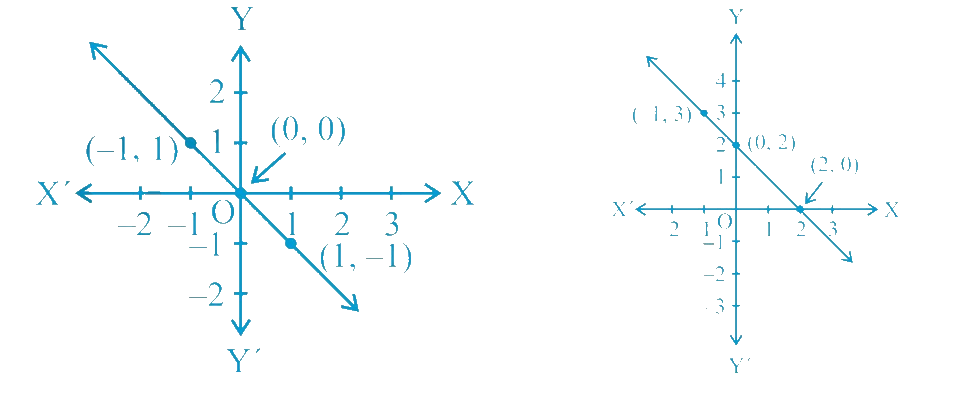### Solution

What is known?

Graph and equations

What is Unknown?

Given equations is of which graph .

Reasoning:

We can check by substituting coordinate values in given equations

Steps:

For the First Figure: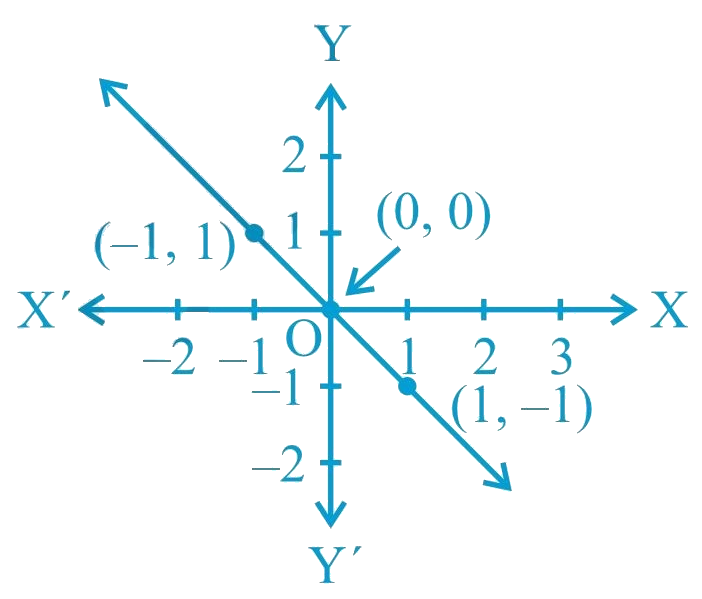• Points on the given line are $$(−1, 1)$$, $$(0, 0),$$ and $$(1, −1)$$.
• It can be observed that the coordinates of the points of the graph satisfy the equation $$x + y = 0$$.

Therefore, $$x + y = 0$$ is the equation corresponding to the graph as shown in the first figure.

Hence, (ii) is the correct answer.

For the Second Figure: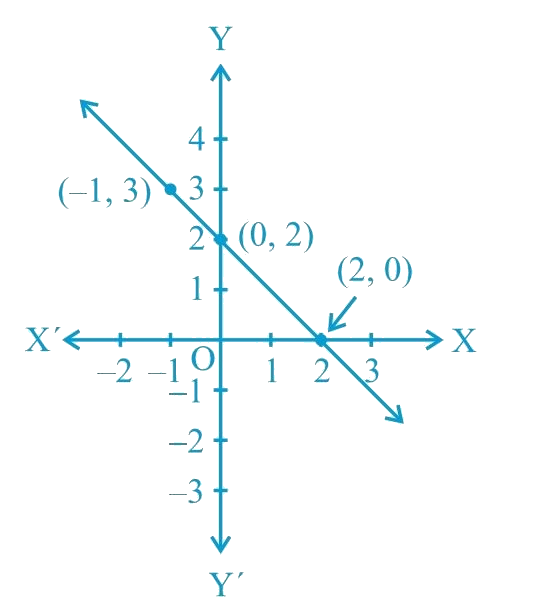• Points on the given line are $$(−1, 3)$$, $$(0, 2),$$ and $$(2, 0).$$
• It can be observed that the coordinates of the points of the graph satisfy the equation $$y = −x + 2$$. Therefore, $$y = −x + 2$$ is the equation corresponding to the graph shown in the second figure.Hence, (iii) is the correct answer.

## Chapter 4 Ex.4.3 Question 6

If the work done by a body on application of a constant force is directly proportional to the distance traveled by the body, express this in the form of an equation in two variables and draw the graph of the same by taking the constant force as 5 units. Also read from the graph the work done when the distance traveled by the body is

(i) $$2 \text { units }$$

(ii) $$0 \text { units }$$

### Solution

What is known?

Force is directly proportional to the distance travelled by the body

What is Unknown?

Formation of linear equation in two variables and graph for it.

Reasoning:

First, we can consider distance travelled and work done as $$x$$ and $$y$$ and then apply the direct proportion property. After forming equation, we can find solution and draw graph for it.

#### Steps:

• Let the distance traveled and the work done by the body be $$x$$ and $$y$$ respectively.
• The constant force applied on the body is $$5\,\rm units.$$
• Work done $$\alpha$$ distance travelled

Hence, $$y$$ $$\alpha$$ $$x$$

$y = kx \qquad \dots \dots \text{ Equation } (1)$

Where, $$k$$ is the constant force applied on the body

Considering the given value of $$k = 5$$ units to solve for $$x$$ and $$y$$

By substituting different values for $$x$$ in the Equation (1) we get different values for $$y$$

• When $$x= 0, y = 0$$
• When $$x= 1, y = 5$$
• When $$x = 2, y= 10$$

Thus, we have the following table with all the obtained solutions:

 $$x$$ $$0$$ $$1$$ $$2$$ $$y$$ $$0$$ $$5$$ $$10$$

By Plotting $$(0, 0) , (1,5)$$ and $$(2, 10)$$ on the graph paper and drawing a line joining them we obtain the required graph

The graph of the line represented by the given equation is as shown.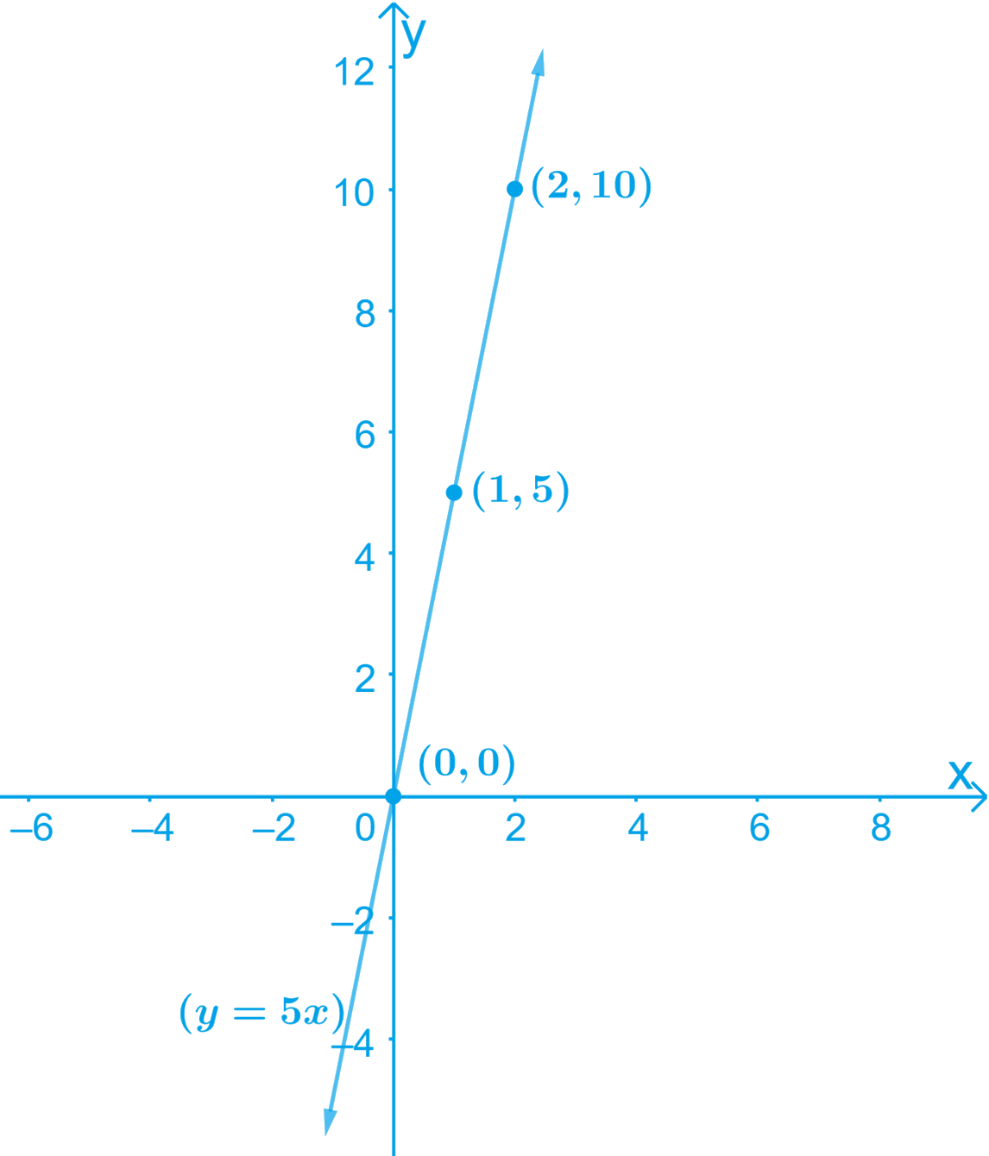(i) From the graphs, it can be observed that the value of $$y$$ corresponding to $$x = 2$$ is $$10\,\rm units.$$This implies that the work done by the body is $$10\,\rm units.$$ when the distance traveled by it is $$2\,\rm units.$$

(ii) From the graphs, it can be observed that the value of $$y$$ corresponding to $$x = 0$$ is $$0.$$ This implies that the work done by the body is $$0\,\rm units$$ when the distance traveled by it is $$0\,\rm units$$

## Chapter 4 Ex.4.3 Question 7

Yamini and Fatima, two students of Class $$IX$$ of a school, together contributed $$₹\, 100$$ towards the Prime Minister’s Relief Fund to help the earthquake victims. Write a linear equation which satisfies this data. (You may take their contributions as ₹ $$x$$ and ₹ $$y$$.) Draw the graph of the same.

#### ### SolutionVideo Solution

What is known?

Yamini and Fatima together contributed $$₹\, 100$$

What is Unknown?

Yamini and Fatima contributions and graph for the same.

Reasoning:

We can let Yamini and Fatima contributions as $$₹$$ $$x$$ and $$₹$$ $$y$$ and form linear equation. Then find $$x$$ and $$y$$ value to draw graph.

Steps:

• Let the amount that Yamini and Fatima have contributed individually be $$₹$$ $$x$$ and $$₹$$ $$y$$ respectively towards the Prime Minister’s Relief fund.
• The total amount contributed by Yamini and Fatima together is $$\rm = ₹\, 100$$

Therefore, $$x + y = 100$$

On transposing, we get $$y = 100 \,– x$$

This is a linear equation in two variables of the form $$ax + by + c = 0$$

Let us consider this as,

$$y = 100\, – x \quad \dots \text{ Equation } (1)$$

By substituting different values of $$x$$ in the Equation (1), we get different values for $$y$$

• When $$x = 0, y = 100$$
• When $$x = 50, y = 50$$
• When $$x = 100, y = 0$$

Thus, we have the following table with all the obtained values of $$x$$ and $$y$$:

 $$x$$ $$0$$ $$50$$ $$100$$ $$y$$ $$100$$ $$50$$ $$0$$

By Plotting the points $$(0, 100), (100, 0)$$ and $$(50, 50)$$ on the graph paper and drawing a line joining them, we obtain the required graph.

The graph of the line represented by the given equation is as shown.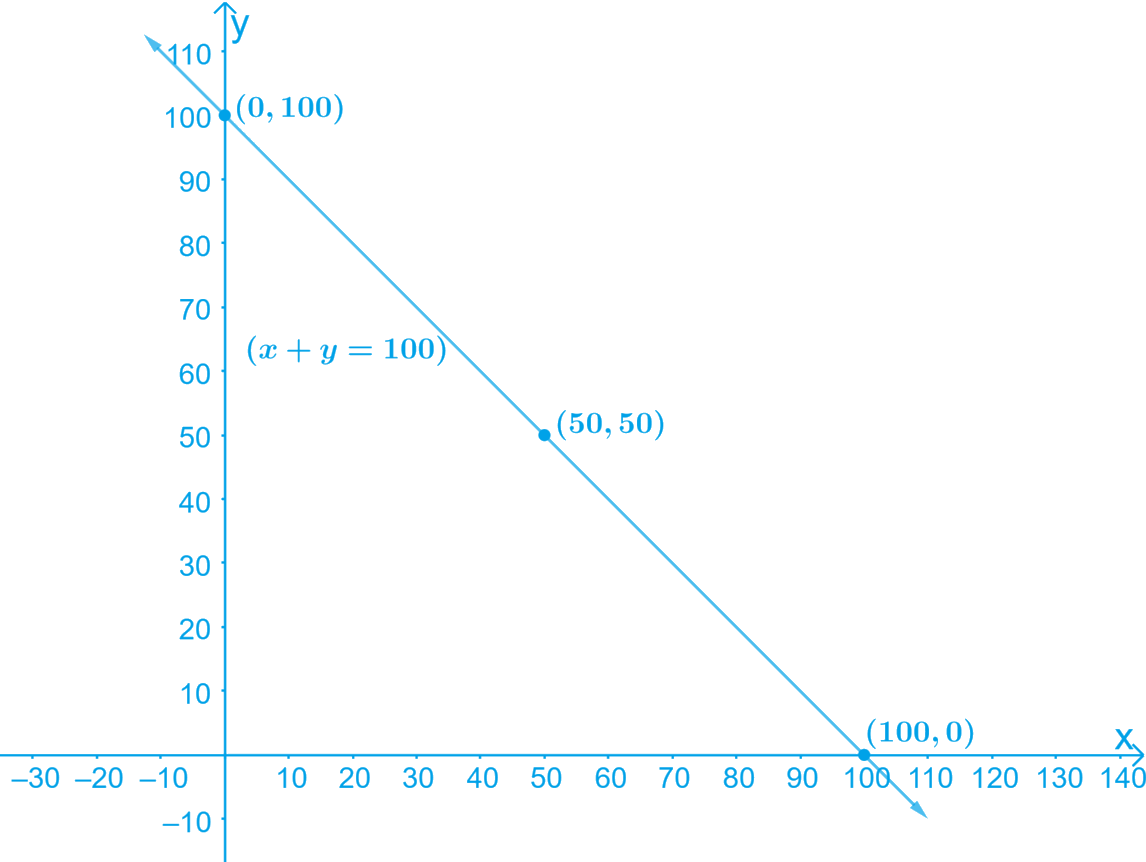Here, variable $$x$$ and $$y$$ are representing the amount contributed by Yamini and Fatima respectively and these quantities cannot be negative. Hence, only those values of $$x$$ and $$y$$ which are lying in the $$1\,\rm st$$ quadrant are considered.

## Chapter 4 Ex.4.3 Question 8

In countries like USA and Canada, the temperature is measured in Fahrenheit, whereas in countries like India, it is measured in Celsius. Here is a linear equation that converts Fahrenheit to Celsius: \begin{align} F = \left( \frac { 9 } { 5 } \right) C + 32 \end{align}

(i) Draw the graph of the linear equation above using Celsius for $$x$$-axis and Fahrenheit for $$y$$-axis.

(ii) If the temperature is $$30^{\circ} C$$, what is the temperature in Fahrenheit?

(iii) If the temperature is $$95^{\circ} F$$, what is the temperature in Celsius?

(iv) If the temperature is $$0^{\circ} C$$, what is the temperature in Fahrenheit and if the temperature is $$0^{\circ} F$$, what is the temperature in Celsius?

(v) Is there a temperature which is numerically the same in both Fahrenheit and Celsius? If yes, find it.

#### ### SolutionVideo Solution

Steps:

(i) Given,

\begin{align} F = \left( \frac { 9 } { 5 } \right) C + 32 \end{align} \dots \text{ Equation } (1)

Equation (1) represents a linear equation of the form $$ax + by + c = 0$$ where $$C$$ and $$F$$ are the two variables.

By substituting different values for $$C$$ in the Equation (1), we obtain different values for $$F$$

When $$C = 0,$$

\begin{align}F = \left( {\frac{9}{5}} \right)C + 32 = \left( {\frac{9}{5}} \right)0 + 32 = 32\end{align}
When $$C = \,– 40,$$

\begin{align}F& = \left( {\frac{9}{5}} \right) \times ( - 40) + 32 \\&= - 72 + 32 = - 40\end{align}
When $$C = 10,$$

\begin{align}F = \left( {\frac{9}{5}} \right) \times (10) + 32 = 18 + 32 = 50\end{align}

Thus, we have the following table with all the obtained solutions:

 $$x$$ $$0$$ $$-40$$ $$10$$ $$y$$ $$32$$ $$-40$$ $$50$$

By Plotting the points $$(– 40, – 40)$$ and $$(10, 50)$$ on the graph point. On joining these points by line segment, we obtain the required graph

The graph of the line represented by the given equation as shown.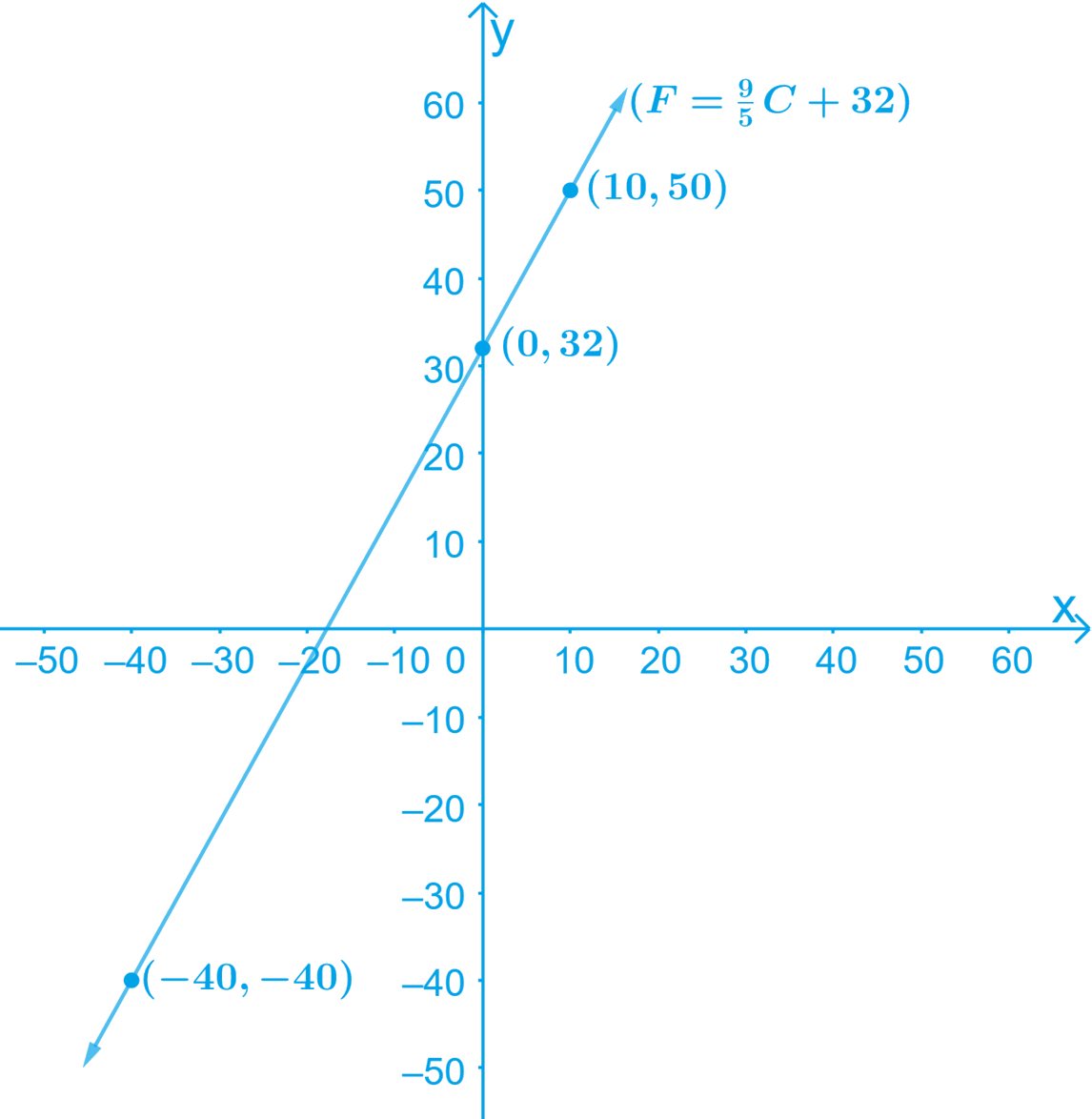(ii) Given:

Temperature $$= 30 ^\circ C$$

To find: $$F=?$$

We know that,\begin{align}F = \left( {\frac{9}{5}} \right)C + 32\end{align}

By Substituting the value of $$C= 30 ^\circ C$$ in the Equation above,

\begin{align}F &= \left( {\frac{9}{5}} \right)C + 32\\ &= \,\left( {\frac{9}{5}} \right)30 + 32\\ &= 54 + 32 \\&= 86 \end{align}

Therefore, the temperature in Fahrenheit is $$86^ {\circ} F$$.

(iii) Given:

Temperature $$=$$ $$95^ {\circ} F$$

To find: $$C= ?$$

We know that,\begin{align} F = \left( \frac { 9 } { 5 } \right) C + 32 \end{align}

By Substituting the value of temperature in the Equation above,

\begin{align}95 &= \left( {\frac{9}{5}} \right)C + 32\\95 - 32 &= \left( {\frac{9}{5}} \right)C\\63 &= \left( {\frac{9}{5}} \right)C\\\therefore C &= \frac{{63 \times 5}}{9} = 35\end{align}

Therefore, the temperature in Celsius is $$35^ {\circ} C$$.

(iv) We know that, \begin{align} F = \left( \frac { 9 } { 5 } \right) C + 32 \end{align}

If $$C = 0^ {\circ}C$$, then by Substituting the value in the above Equation,

\begin{align}F &= \left( {\frac{9}{5}} \right)0 + 32\\F &= 0 + 32\\F &= 32\end{align}

Therefore, if $$C = 0^ {\circ}C$$, then $$F = 32^ {\circ}F$$.

If $$F = 0^ {\circ}F$$, then by Substituting the value in the above Equation,

\begin{align}0 &= \left( {\frac{9}{5}} \right)C + 32\\\left( {\frac{9}{5}} \right)C &= - 32\\C &= \frac{{ - 32 \times 5}}{9} = - 17.77\end{align}

Therefore, if $$F = 0^ {\circ}F$$, then $$C = 17.8^ {\circ}C$$.

(v) We know that,

\begin{align} F = \left( \frac { 9 } { 5 } \right) C + 32 \end{align}

Let us consider, $$F = C$$

By Substituting this value in the Equation above,

\begin{align}&F = \left( {\frac{9}{5}} \right)F + 32\\&\left( {\frac{9}{5} - 1} \right)F + 32 = 0\\&\left( {\frac{4}{5}} \right)F = - 32\\&F = \frac{{ - 32 \times 5}}{4}\end{align}

Hence, $$F = \,–40^ {\circ}F$$

Yes, there is a temperature, $$−40^ {\circ}F$$, which is numerically the same in both Fahrenheit and Celsius.

Related Sections
Related Sections
Instant doubt clearing with Cuemath Advanced Math Program